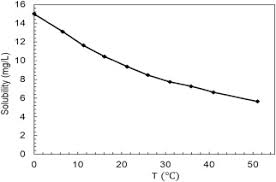## How to Calculate and Solve for Marhoun’s Gas Solubility in a Fluid | The Calculator EncyclopediaThe image above represents marhoun’s gas solubility.

To compute for the marhoun’s gas solubility, four essential parameters are needed and these parameters are Gas Specific Gravity (γg), Temperature (°Rankine) (T), Pressure (P) and Stock Tank Oil Gravity (γo).

The formula for calculating the marhoun’s gas solubility:

Rs = [a γgb γoc Td P]e

Where:

Rs = Marhoun’s Gas Solubility
a = 185.843208
b = 1.877840
c = -3.1437
d = -1.32657
e = 1.398441
γg = Gas Specific Gravity
T = Temperature (°Rankine)
P = Pressure
γo = Stock Tank Oil Gravity

Let’s solve an example;
Find the marhoun’s gas solubility when the gas specific gravity is 14, temperature is 80, pressure is 40 and the stock tank oil gravity is 24.

This implies that;

γg = Gas Specific Gravity = 14
T = Temperature (°Rankine) = 80
P = Pressure = 40
γo = Stock Tank Oil Gravity = 24

Rs = [a γgb γoc Td P]e
Rs = [185.843208 x 141.87784 x 24-3.1437 x 80-1.32657 x 40]1.398441
Rs = [185.843208 x 141.98 x 24-3.1437 x 80-1.32657 x 40]1.398441
Rs = [185.843208 x 141.98 x 0.0000458 x 80-1.32657 x 40]1.398441
Rs = [185.843208 x 141.98 x 0.0000458 x 0.00298 x 40]1.398441
Rs = [0.1445]1.398441
Rs = 0.0668

Therefore, the marhoun’s gas solubility is 0.0668.